# Energy and its Types

Energy

The energy of a body is defined as its capacity for doing work.

(1) Since energy of a body is the total quantity of work done therefore it is a scalar quantity.

(2) Dimension: ML2T-2 it is same as that of work or torque.

(3) Units    : Joule [S.I.], erg [C.G.S.]

Practical units : electron volt (eV), Kilowatt hour (KWh), Calories (Cal)

Relation between different units:

1 Joule = 107erg

1 eV  = 1.6 * 10-19Joule

1 KWh = 3.6*106Joule

1 Calorie = 4.18 Joule

Types of Energy

1. Kinetic Energy
2. Potential Energy
3. Electrostatic Energy
4. Magnetic Energy
5. Heat Energy
6. Electrical Energy
7. Nuclear Energy

Etc……….

Here we are going to study  Mechanical Energy which is equal to the sum of Kinetic Energy and Potential Energy.

Kinetic Energy:  It is the internal capacity to do work by virtue of relative motion.

It is the energy associated with the body by virtue of its motion.

->Kinetic Energy is a scalar quantity.

->It is a relative quantity.

->Its unit and dimensions are same as that of Energy.

-> It can never be negative.

->K.E= (1/2)mv2

Relation Between Kinetic Energy and Momentum

K=(1/2)mv2

p=mv

v=p/m

put this value of v in formula of kinetic energy

K=(1/2)m(p/m)2  =  p2/2m

Potential Energy is the energy associated with an object by virtue of its configuration.

->It is a scalar quantity

->Its unit and dimensions are same as that of Energy.

->It can be negative ,positive or zero.

->Its value depends upon a reference.

Types of Potential Energy

1. Gravitational Potential Energy
2. Elastic Potential Energy

Gravitational Potential Energy-> It is the energy possessed by the object by virtue of its position with respect to earth (earth is taken as reference).

P.E= mgh

Elastic Potential Energy is the energy possessed by an elastic body  by virtue of expansion or compression.

Restoring force and spring constant : When a spring is stretched or compressed from its normal position (x = 0) by a small distance x, then a restoring force is produced in the spring to bring it to the normal position.

According to Hooke’s law this restoring force is proportional to the displacement x and its direction is always opposite to the displacement.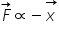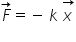where k is called spring constant.

If x = 1, F = k (Numerically)

or                               k = F

Hence spring constant is numerically equal to force required to produce unit displacement (compression or extension) in the spring. If required force is more, then spring is said to be more stiff and vice-versa.

Actually k is a measure of the stiffness/softness of the spring.

Dimensions =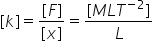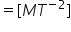Units :  S.I. unit Newton/metre, C.G.S unit Dyne/cm.

Expression for elastic potential energy : When a spring is stretched or compressed from its normal position (x = 0), work has to be done by external force against restoring force.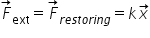Let the spring is further stretched through the distance dx, then work done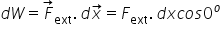=kx dx

Therefore total work done to stretch the spring through a distance x from its mean position is given by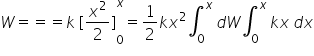This work done is stored as the potential energy of the stretched spring.

therefore Elastic potential energy= U= (1/2)kx2

Note: If spring is stretched from initial position x1 to final position x2 then work done

= Increment in elastic potential energy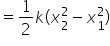Graph of Energy vs position

Related Keywords